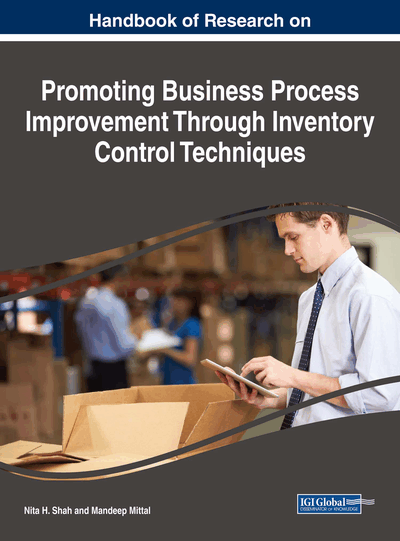# Imprecise Inventory Model for Items With Imperfect Quality Subject to Learning Effects Having Shortages

Sumana Saha (Calcutta University, India) and Tripti Chakrabarti (Calcutta University, India)
DOI: 10.4018/978-1-5225-3232-3.ch016
Available
\$37.50
No Current Special Offers

## Abstract

The fundamental assumption of an economic order quantity (EOQ) model is that 100% of items in an ordered lot are perfect. This assumption is not always pertinent for production processes because of process deterioration or other factors. This paper develops an EOQ model for that each ordered lot contains some defective items and shortages backordered. Here, an inventory model is developed to deal the impreciseness present in market demand. It is assumed that the received items are not of perfect quality and after screening, imperfect items are withdrawn from inventory and sold at discounted price. However, in practice, errors occur in screening test. So, the screening process fails to be perfect. Due to acquaintance with handling methodology and system, holding cost and ordering cost are gradually decreases from one shipment to another. So, learning effect is incorporated on holding cost, ordering cost and number of defective items present in each lot. Due to impreciseness in market demand and in different inventory costs, profit expression is fuzzy in nature. To fuzzify the profit expression, Extension Principle is used and for defuzzification Signed distance method is applied. Finally, the feasibility of proposed model and the effect of learning on optimal solution are shown through numerical example.
Chapter Preview
Top

## Introduction

Economic order quantity model (EOQ) is a technique to find out optimum order quantity generally by considering costs of procurement, inventory holding, and backorder. The basic assumption of the classical EOQ model is that 100% of ordered items are perfect. This assumption may not be valid for most of the production environments. Starting from this point, researchers have developed different EOQ and economic production quantity (EPQ) models with percentage defective items. Rosenblatt and Lee (1986) proposed an EPQ model for a production system which contains defective production. The basic assumption in their model is that the production system produces 100% non-defective products from the starting point of production until a time point which is a random variable. At this time point, system becomes out of control and starts to produce defective items with a percentage of production until end of the production period. It is assumed that the distribution of time passes until system becomes out of control state is exponential. Backorder is not allowed in their model. Chung and Hou (2003) combined aforementioned models by allowing assumption of backorders. Salameh and Jaber (2000) developed an EOQ model for circumstances where a fraction of the ordered lot is of imperfect quality and has a uniform distribution. Their model assumed that shortages are not permitted to occur. In many real-life conditions, stockout is unavoidable because of various uncertainties in the related system. Therefore, the occurrence of shortages in inventory could be considered as a natural phenomenon.

Several researchers studied inventory models with market demand as crisp, stochastic or fuzzy parameter. Chen and Wang (1996) fuzzified the order cost, inventory cost and backorder cost into trapezoidal fuzzy numbers and used the functional principle to obtain the estimate of the total cost in the fuzzy sense. In Gen et al. (1997), they considered the fuzzy input data expressed by fuzzy numbers and the interval mean value concept. Yao et al. (2003) discussed inventory problems in the fuzzy sense. Salameh and Jaber (2000)Jaber and Bonney (2003), Papachristos and Konstantaras (2006) developed the inventory model by taking market demand as crisp parameters. It is observed that in practical situations, crisp market demand can be justified only for the maturity phase of the product. stochastic market demand is applicable only for old product where probability distribution function can be determined by past data. For many products like clothes, fashion accessories, mobile phones etc., the market demand cannot be predicted accurately and the probability distribution function cannot be determined. To develop the inventory models of such products, a major difficulty faced by researchers is to forecast the demand. It is not possible for researchers to decide the exact market demand in such a complex, changing and uncertain environment. So, it is often found that market demand is imprecise in nature.

## Complete Chapter List

Search this Book:
Reset